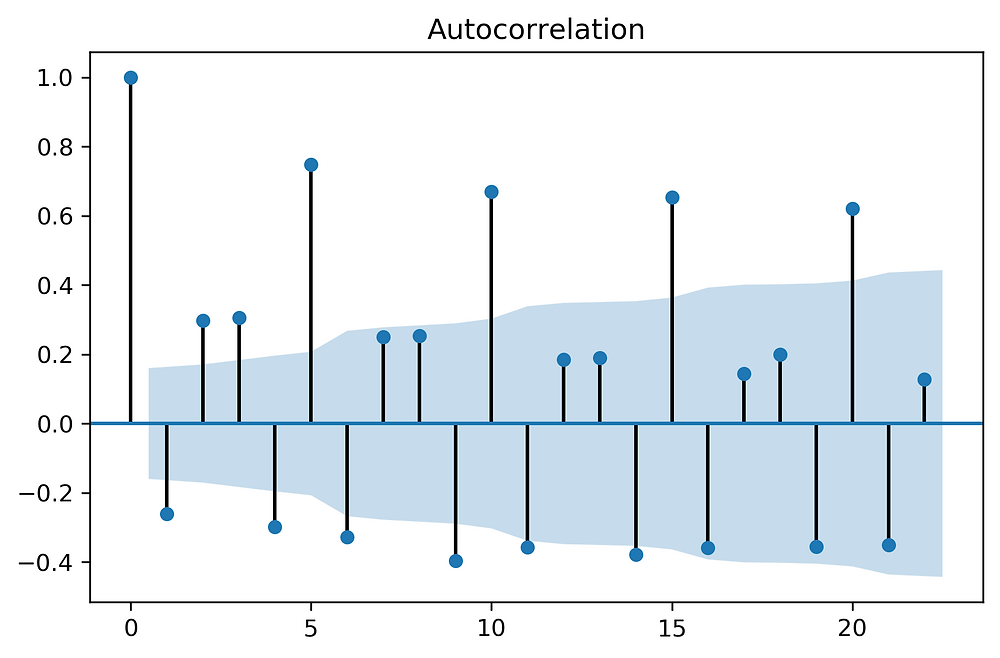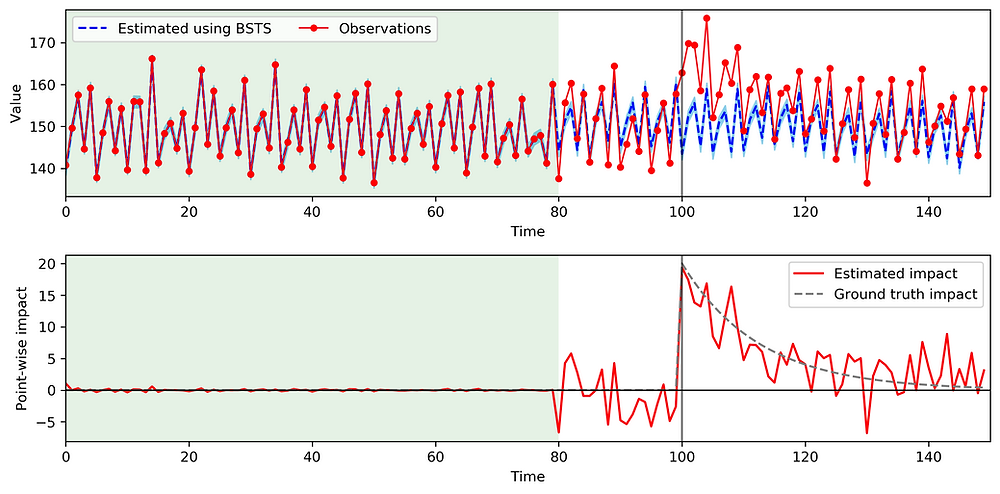Search
•Taka Yabe

# Pystan - Causal inference using Bayesian Structural Time Series

Updated: Feb 21, 2021

** The objective of this post is to introduce the Pystan implementation of BSTS **

You can see my online seminar on using BSTS for "estimating the causal impact of disasters to business firms" at PlaceKey Research Seminar (video).

## Causal inference on time series data

Time series data are becoming more and more common thanks to various large scale sensor systems, including mobile phone /smartphone networks and social media platforms, which I mainly work with in my research.

There are many instances where we want to assess the impact of some treatment on time series data, which we will refer to as causal inference. Examples include:

• "what was the effect of an ad on click through rates (CTRs) on a website"

## Intro to Bayesian structural time series

Bayesian structural time series (BSTS) model is a statistical technique used for feature selection, time series forecasting, nowcasting, inferring causal impact and other applications. The model is designed to work with time series data. (wikipedia)

Other causal inference approaches include:

• Difference in differences models (common in Economics)

• Interrupted time series designs

The advantages of BSTS are that we are able to:

• Infer the temporal dynamics of the impact caused by the treatment

• Impose prior distributions on model parameters (Bayesian approach)

• Very flexible (you can design various sources of variation, including time-varying covariates, seasonality trends etc.)

There are several libraries for BSTS that are easy to use, written in R:

Although these were very easy to use,

1. I wanted more flexibility in my code and

2. I wanted to model using Python, which I am more familiar with

## Mathematical formulation of BSTS

The set of equations below shows one example of the BSTS model.

• Equation 1: The observation y_t, is modeled as the linear sum of the local trend \mu_t, seasonality \tau_t (S denotes the cycle; if we want to model the weekly trend, we set S=6), covariate effect \beta_t x_t , and an error term \epsilon_t.

• The following equations 2,3,4 show the temporal evolution of each component, and the rest show the prior distribution functions for the error term of each model parameter.

• The bottom line shows the hyper-priors for the standard deviations of the error terms, which are modeled as a Half-Cauchy here.The graphic below shows how the variables evolve over time. According to the problem setting and data you are interested in, all of this architecture can be changed around, which is a great (and fun) property of BSTS.## Implementation using Python + Pystan

### Pystan

Here, we will implement the BSTS using Python, more specifically, pystan, which is a Python interface to stan, which is a package for Bayesian computation. pystan can be installed using the following command:

python3 -m pip install pystan

There are many tutorials on using pystan for Bayesian inference, so please refer to them if you are not familiar:

We'll go right into implementing BSTS here.

### BSTS using Pystan

Import libraries including pystan

import numpy as np
import pandas as pd
import matplotlib.pyplot as plt
from matplotlib.gridspec import GridSpec
from scipy.stats import pearsonr
import time
import pystan

The stan code is composed of "data", "parameters", "transformed parameters", "model", and "generated quantities" (if we're making predictions beyond the pre-treatment period).

The example here uses:

• Cauchy(0,1) for priors, and

• 5-step seasonality (this should be determined by looking at the auto-correlation of the time series data).

• Gaussian assumption for likelihood

stan_code = """
data {
int<lower=1> T; // Number of timesteps
int<lower=1> T_forecast;
vector[T] y; // observed value
vector[T+T_forecast] x; // covariate
}

parameters {
real<lower=0> sigma_mu;
real<lower=0> sigma_tau;
real<lower=0> sigma_y;
vector[T] mu_err;
vector[T] tau_err;
real beta;
}

transformed parameters {
vector[T] mu; // local trend
vector[T] tau; // seasonality
mu = mu_err;
tau = tau_err;
tau = tau_err;
tau = tau_err;
tau = tau_err;
for(t in 5:T){
tau[t] = -(tau[t-1]+tau[t-2]+tau[t-3]+tau[t-4]) + tau_err[t];
}
for(t in 2:T){
mu[t] = mu[t-1] + mu_err[t];
}
}

model {
// Priors
sigma_mu ~ cauchy(0,1);
sigma_tau ~ cauchy(0,1);
sigma_y ~ cauchy(0,1);
beta ~ cauchy(0,1);
mu_err ~ normal(0,sigma_mu);
tau_err ~ normal(0,sigma_tau);

for(t in 1:T){
y[t] ~ normal(mu[t] + tau[t], sigma_y);
}
}

// for post-intervention predictions
generated quantities {
real y_forecast[T_forecast];
real mu_forecast[T_forecast];
real tau_forecast[T_forecast];
mu_forecast = normal_rng(mu[T], sigma_mu);
for (t in 2:T_forecast) {
mu_forecast[t] = normal_rng(mu_forecast[t-1], sigma_mu);
}

tau_forecast = normal_rng(-(tau[T]+tau[T-1]+tau[T-2]+tau[T-3]), sigma_tau);
tau_forecast = normal_rng(-(tau[T]+tau[T-1]+tau[T-2]+tau_forecast), sigma_tau);
tau_forecast = normal_rng(-(tau[T]+tau[T-1]+tau_forecast+tau_forecast), sigma_tau);
tau_forecast = normal_rng(-(tau[T]+tau_forecast+tau_forecast+tau_forecast), sigma_tau);
for (t in 5:T_forecast) {
tau_forecast[t] = normal_rng(-(tau_forecast[t-1]+tau_forecast[t-2]+tau_forecast[t-3]+tau_forecast[t-4]), sigma_tau);
}
for (t in 1:T_forecast) {
y_forecast[t] = normal_rng(mu_forecast[t] + tau_forecast[t], sigma_y);
}
}
"""

Some tips:

• Push \mu_t, \tau_t inside "transformed parameters" to make code compact

• T= timesteps in training period; T_forecast = timesteps for post-treatment prediction

• use normal_rng function for prediction!

## Experiment using synthetic data

Generate synthetic data.

In this example, we assume no covariate effect for simplicity. The time series is linear combination of a Gaussian random variable + 5-cycle seasonality, and treatment (exponential treatment starting at T=100).

x = np.zeros(150)
beta = 1
mu = np.random.normal(150, 1, 150)
tau = np.tile(np.asarray([-10,0,5,-5,10]),30)
treatment = np.concatenate((np.zeros(100),20*np.exp(-0.08*np.arange(50))))
y = mu+tau+treatment+x*beta

Plot synthetic data:

fig,ax = plt.subplots(figsize=(10,3))
ax.plot(y)
plt.show()You can easily check the temporal auto-correlation to determine your seasonality term:

from statsmodels.graphics.tsaplots import plot_acf
plot_acf(y)
plt.show()We can see that this time series has a seasonality of S=5.

Data input:

data_input = {'T': 80,
'T_forecast': 70,
'x': x,
'y': y[:80]
}

Define model:

sm = pystan.StanModel(model_code=stan_code)

Run pystan sampling: (number of iterations, chains, and warmup steps should be decided based on the model fit.)

fit = sm.sampling(data=data_input, iter=5000, chains=1, warmup=1000, refresh=500)

Look at the summary of estimation, and check that Rhat < 1.1 for good mixing

print(fit)Process and plot estimations against the ground truth data

mu = fit["mu"]
ta = fit["tau"]
beta = fit["beta"]
y_forecast = fit["y_forecast"]
sigma_y = np.median(fit["sigma_y"])
mu_mean = np.median(mu, axis=0)
ta_mean = np.median(ta, axis=0)
beta_mean = np.median(beta, axis=0)
y_mean  = mu_mean+ta_mean +beta_mean*x[:80]
y_forecast_mean  = np.median(y_forecast, axis=0)
y_all = np.concatenate((y_mean,  y_forecast_mean))
fig = plt.figure(figsize=(10,5))
gs=GridSpec(2,1)

ax1.plot(y_all, color="blue", label="Estimated using BSTS", linestyle="--")
ax1.plot(y, color="r", marker ="o", markersize=4, label="Observations", linewidth=1, alpha=1)
ax1.fill_between(np.arange(len(y_all)), y_all+1*sigma_y, y_all-1*sigma_y, color="skyblue")
ax1.axvspan(0, 80, facecolor='green', alpha=0.1)
ax1.axvline(100, color='k', alpha=0.5)
ax1.legend(ncol=2)
ax1.set_ylabel("Value")
ax1.set_xlabel("Time")
ax1.set_xlim(0,150)

ax2.plot(y-y_all, color="red", label="Estimated impact")
ax2.plot(treatment, color="gray", linestyle="--", label="Ground truth impact")
ax2.axhline(0, color="k", linewidth=1)
ax2.axvspan(0, 80, facecolor='green', alpha=0.1)
ax2.axvline(100, color='k', alpha=0.5)
ax2.legend()
ax2.set_ylabel("Point-wise impact")
ax2.set_xlabel("Time")
ax2.set_xlim(0,150)

plt.tight_layout()
plt.savefig('.../syntheticres.png',dpi=300,bbox_inches="tight")
plt.show()Bottom panel shows that the estimated impact has a good match with ground truth impact.

Changing the model parameters (prior distribution parameters, model structure, etc.) could further improve the predictions and adjust to more complex data.

## Example with real world data

In short, check out our paper:

"Quantifying the economic impact of disasters on businesses using human mobility data: a Bayesian causal inference approach", Yabe, Takahiro, Yunchang Zhang, and Satish V. Ukkusuri. EPJ Data Science 9, no. 1 (2020): 36.

In recent years, extreme shocks, such as natural disasters, are increasing in both frequency and intensity, causing significant economic loss to many cities around the world. Quantifying the economic cost of local businesses after extreme shocks is important for post-disaster assessment and pre-disaster planning. Conventionally, surveys have been the primary source of data used to quantify damages inflicted on businesses by disasters. However, surveys often suffer from high cost and long time for implementation, spatio-temporal sparsity in observations, and limitations in scalability.

Recently, large scale human mobility data (e.g. mobile phone GPS) have been used to observe and analyze human mobility patterns in an unprecedented spatio-temporal granularity and scale. In this work, we use location data collected from mobile phones to estimate and analyze the causal impact of hurricanes on business performance.

To quantify the causal impact of the disaster, we use a Bayesian structural time series model to predict the counterfactual performances of affected businesses (what if the disaster did not occur?), which may use performances of other businesses outside the disaster areas as covariates. The method is tested to quantify the resilience of 635 businesses across 9 categories in Puerto Rico after Hurricane Maria.

Example with real world mobility data from Puerto Rico for a Walmart:We further conducted analysis of the impacts of the following factors on disaster impacts

• industry category of business (NAICS code)

For more details, here is a video recording of the presentation: